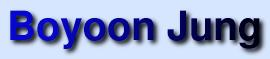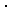Boyoon Jung's Library Manual

# bj::MDP Class Reference [Markov Decision Process]

A Markov Decision Process Solver. More...

`#include <mdp.h>`

List of all members.

## Public Member Functions

MDP (unsigned int nstates, unsigned int nactions, double *T=0, double *R=0)
A constructor.
~MDP (void)
A destructor.
void initialize (double *T, double *R)
Initialize MDP models.
unsigned int * value_iteration (double discount=0.9, double epsilon=0.01, double *v0=0, int max_iter=-1)
Value iteration algorithm.
unsigned int * policy_iteration (double discount=0.9, double *p0=0, int max_iter=1000)
Policy iteration algorithm.
unsigned int * policy (void)
Return an optimal policy.
double * value_function (void)
Return a value function.
double getT (unsigned int s, unsigned int a, unsigned int sp)
Get an element of a transition probability matrix.
void setT (unsigned int s, unsigned int a, unsigned int sp, double v)
Set the value of a transition probability matrix.
double getR (unsigned int s, unsigned int a)
Get an element of a reward matrix.
void setR (unsigned int s, unsigned int a, double v)
Set the value of a reward matrix.
unsigned int numStates (void)
Return the number of states.
unsigned int numActions (void)
Return the number of actions.

## Static Public Member Functions

void convert_S_SA (unsigned int nstates, unsigned int nactions, double *t, double *s, double *sa)
Convert a state-only reward matrix into a state-action matrix.
void convert_SAS_SA (unsigned int nstates, unsigned int nactions, double *t, double *sas, double *sa)
Convert a state-aciton-state reward matrix into a state-action matrix.

## Protected Member Functions

virtual void load_transition_matrix (void)
Load a transition probability matrix.
virtual void load_reward_matrix (void)
Load a reward matrix.
void bellman_operation (unsigned int *p, double *v, double *t, double *r, double *vt_1, double discount)
Apply the bellman operation.
void policy_evaluation (double *v, double *t, double *r, unsigned int *p, double discount)
Evaluate a policy.
double span_semi_norm (double *vt, double *vt_1, unsigned int size)
Compute the span semi-norm.
bool match_policy (unsigned int *p0, unsigned int *p1, unsigned int size)
Check if two policies are matched.
void array_swap (double *&a0, double *&a1)
Swap two arrays.
void array_swap (unsigned int *&a0, unsigned int *&a1)
Swap two arrays.
double array_max (double *a, unsigned int size, unsigned int &index)
Find a maximum value in an array.
double sub_matrix_min (double *a, unsigned int rows, unsigned int columns, unsigned int index)
Find a minimum sub-matrix.

## Protected Attributes

int num_states
The size of state space.
int num_actions
The number of actions.
double * T
A transition probability matrix.
double * R
A reward matrix.
unsigned int * P
An optimal policy.
double * V
A value function.
double * Vt_1
A value function in the previous step.
double * Q
An action-value function.

## Detailed Description

A Markov Decision Process Solver.

MDP class provides a MDP (Markov Decision Process) solver. It provides both value iteration and policy iteration algorithms. Since a transition probability matrix and a reward matrix are stored in multi-dimentional array form, the required memory size is huge.

## Constructor & Destructor Documentation

 bj::MDP::MDP ( unsigned int nstates, unsigned int nactions, double * T = `0`, double * R = `0` )

A constructor.

Parameters:
 nstates The number of states. nactions The number of actions. T A transition probability matrix. If 0, load_transition_matrix() should be overloaded by an inheriting class. R A reward matrix. If 0, load_reward_matrix() should be overloaded by an inheriting class.

 bj::MDP::~MDP ( void )
 A destructor.

## Member Function Documentation

 double bj::MDP::array_max ( double * a, unsigned int size, unsigned int & index ) ` [inline, protected]`
 Find a maximum value in an array.

 void bj::MDP::array_swap ( unsigned int *& a0, unsigned int *& a1 ) ` [inline, protected]`
 Swap two arrays.

 void bj::MDP::array_swap ( double *& a0, double *& a1 ) ` [inline, protected]`
 Swap two arrays.

 void bj::MDP::bellman_operation ( unsigned int * p, double * v, double * t, double * r, double * vt_1, double discount ) ` [inline, protected]`
 Apply the bellman operation.

 void bj::MDP::convert_S_SA ( unsigned int nstates, unsigned int nactions, double * t, double * s, double * sa ) ` [static]`

Convert a state-only reward matrix into a state-action matrix.

Parameters:
 nstates The number of states. nactions The number of actions. t A transition probability matrix. s A state-only reward matrix. sa A state-action reward matrix.

 void bj::MDP::convert_SAS_SA ( unsigned int nstates, unsigned int nactions, double * t, double * sas, double * sa ) ` [static]`

Convert a state-aciton-state reward matrix into a state-action matrix.

Parameters:
 nstates The number of states. nactions The number of actions. t A transition probability matrix. sas A state-action-state reward matrix. sa A state-action reward matrix.

 double bj::MDP::getR ( unsigned int s, unsigned int a ) ` [inline]`
 Get an element of a reward matrix.

 double bj::MDP::getT ( unsigned int s, unsigned int a, unsigned int sp ) ` [inline]`
 Get an element of a transition probability matrix.

 void bj::MDP::initialize ( double * T, double * R )

Initialize MDP models.

Parameters:
 T A transition probability matrix. R A reward matrix.

 virtual void bj::MDP::load_reward_matrix ( void ) ` [inline, protected, virtual]`
 Load a reward matrix. This function should be overloaded by an inheriting class unless a reward matrix R is provided in a constructor.

 virtual void bj::MDP::load_transition_matrix ( void ) ` [inline, protected, virtual]`
 Load a transition probability matrix. This function should be overloaded by an inheriting class unless a transition probability matrix T is provided in a constructor.

 bool bj::MDP::match_policy ( unsigned int * p0, unsigned int * p1, unsigned int size ) ` [inline, protected]`
 Check if two policies are matched.

 unsigned int bj::MDP::numActions ( void ) ` [inline]`
 Return the number of actions.

 unsigned int bj::MDP::numStates ( void ) ` [inline]`
 Return the number of states.

 unsigned int* bj::MDP::policy ( void ) ` [inline]`
 Return an optimal policy.

 void bj::MDP::policy_evaluation ( double * v, double * t, double * r, unsigned int * p, double discount ) ` [protected]`
 Evaluate a policy.

 unsigned int* bj::MDP::policy_iteration ( double discount = `0.9`, double * p0 = `0`, int max_iter = `1000` )

Policy iteration algorithm.

Parameters:
 discount A discount factor. [0 ~ 1] p0 An initial policy. max_iter Maximum number of iterations.

 void bj::MDP::setR ( unsigned int s, unsigned int a, double v ) ` [inline]`
 Set the value of a reward matrix.

 void bj::MDP::setT ( unsigned int s, unsigned int a, unsigned int sp, double v ) ` [inline]`
 Set the value of a transition probability matrix.

 double bj::MDP::span_semi_norm ( double * vt, double * vt_1, unsigned int size ) ` [inline, protected]`
 Compute the span semi-norm.

 double bj::MDP::sub_matrix_min ( double * a, unsigned int rows, unsigned int columns, unsigned int index ) ` [inline, protected]`
 Find a minimum sub-matrix.

 double* bj::MDP::value_function ( void ) ` [inline]`
 Return a value function.

 unsigned int* bj::MDP::value_iteration ( double discount = `0.9`, double epsilon = `0.01`, double * v0 = `0`, int max_iter = `-1` )

Value iteration algorithm.

Parameters:
 discount A discount factor. [0 ~ 1] epsilon Search of an optimal policy. ]0 ~ 1] v0 An initial value function. max_iter Maximum number of iterations. If negative, a proper number of iterations is computed.

## Member Data Documentation

 int bj::MDP::num_actions` [protected]`
 The number of actions.

 int bj::MDP::num_states` [protected]`
 The size of state space.

 unsigned int* bj::MDP::P` [protected]`
 An optimal policy.

 double* bj::MDP::Q` [protected]`
 An action-value function.

 double* bj::MDP::R` [protected]`
 A reward matrix.

 double* bj::MDP::T` [protected]`
 A transition probability matrix.

 double* bj::MDP::V` [protected]`
 A value function.

 double* bj::MDP::Vt_1` [protected]`
 A value function in the previous step.

The documentation for this class was generated from the following file:
ResearchProjectsPublications

ToolsBjLib ManualPlayer/StagePyPlayerMicroStrain IMUGPS/DGPSParticle Filter

PersonalWelcomeCurriculum VitaeResumeContact

Outside LinksUSCUSC CS DeptCRESRobotics LabRESL